# What's behind binomial coefficients?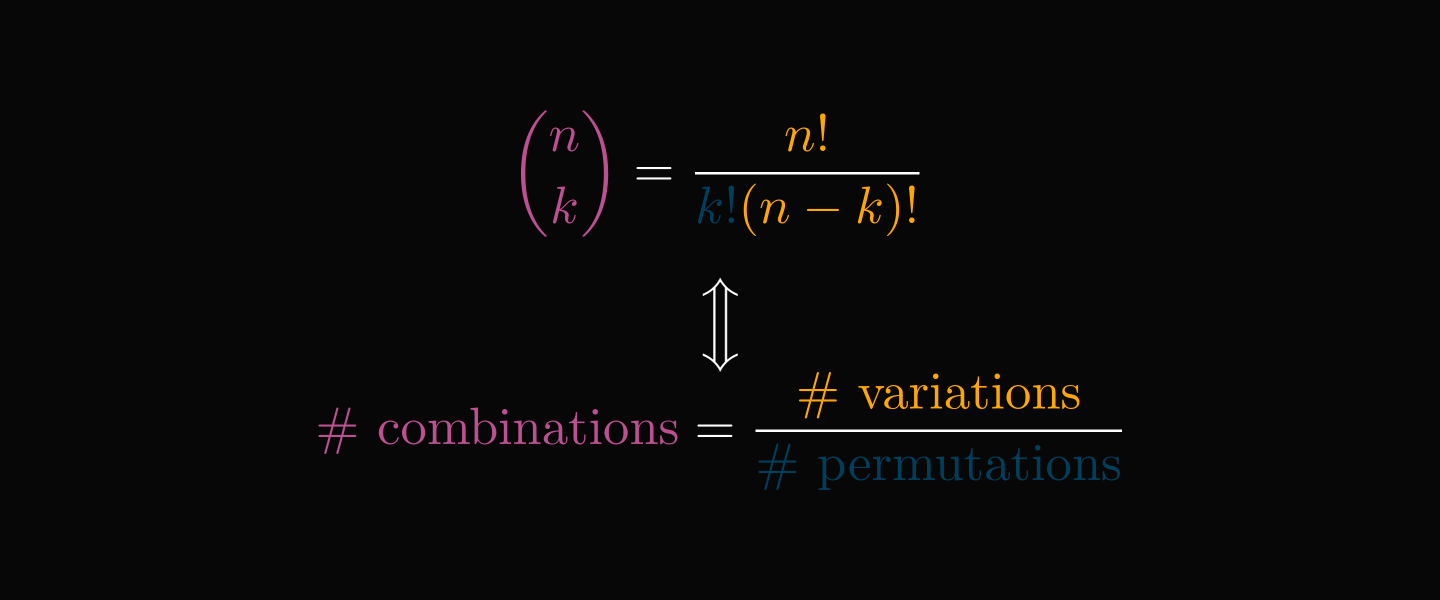Binomial coefficients are one of the most ubiquitous quantities in mathematics, used in every field from combinatorics to probability.

Behind their formula, there is a principle that reveals a deep connection between combinations, variations, and permutations.

Let's explore it!

By definition, the binomial coefficients describe the number of ways one can select $\textstyle k$ elements from a set of $\textstyle n$ elements:

\begin{align*} \binom{n}{k} = \big| \big\{ S \subseteq \{1, 2, \dots, n\}: |S| = k \big\} \big|. \end{align*}

How does this definition yield the famous factorial formula? We'll see a special case to shed some light on the question!

Suppose that we have a set of 5 elements and want to know how many of its subsets have exactly 3 elements. A subset is called a combination, and the order of elements doesn't matter.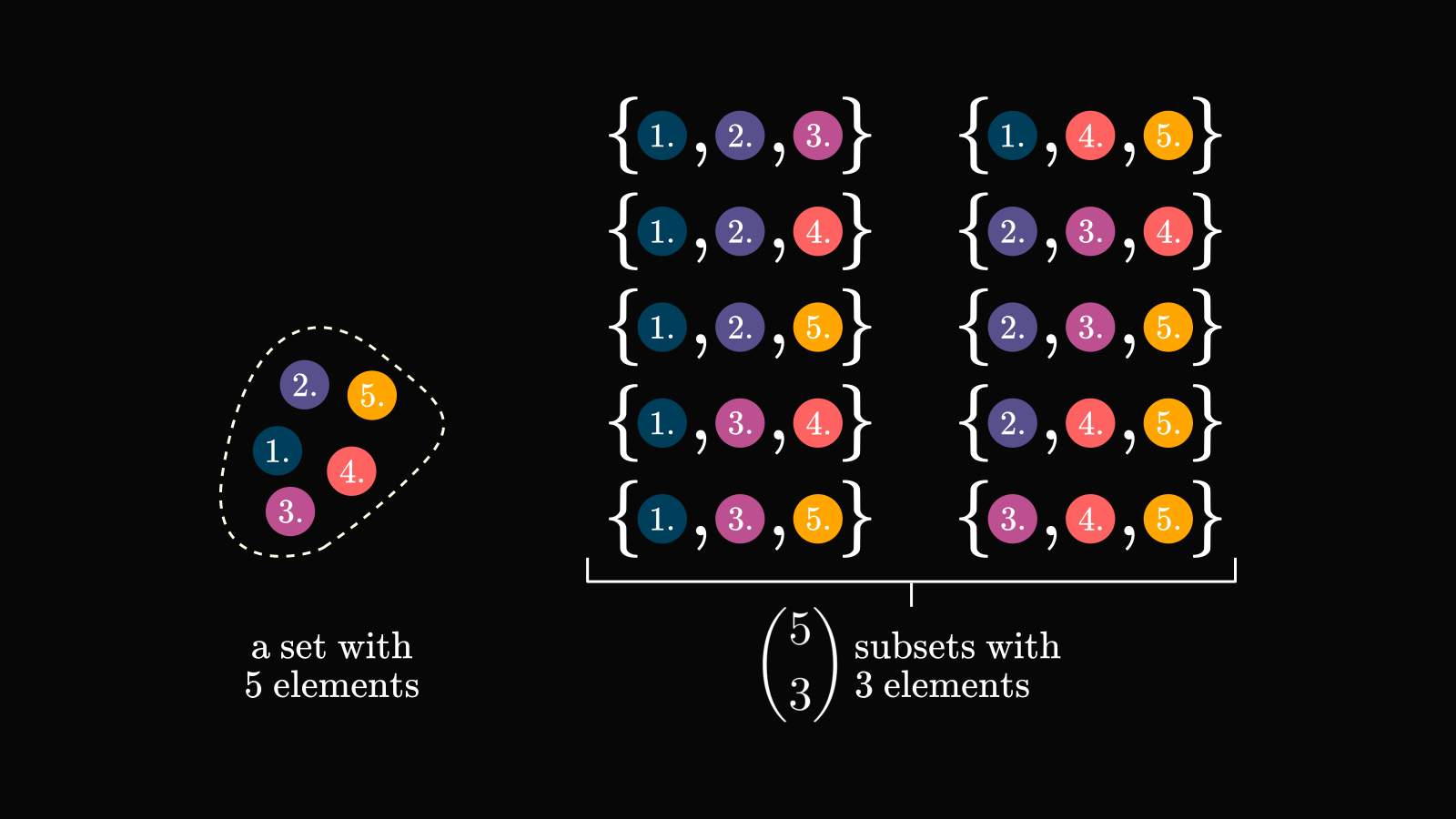Counting combinations seems complicated, so let's make the problem a bit more special: let's count the 3-element tuples, where the ordering is important! Such a tuple is called a variation.

There are $5 \cdot 4 \cdot 3$ possible variations, as we have

• 5 options for the 1st position,
• 4 remaining options for the 2nd position,
• and 3 remaining options for the 3rd position.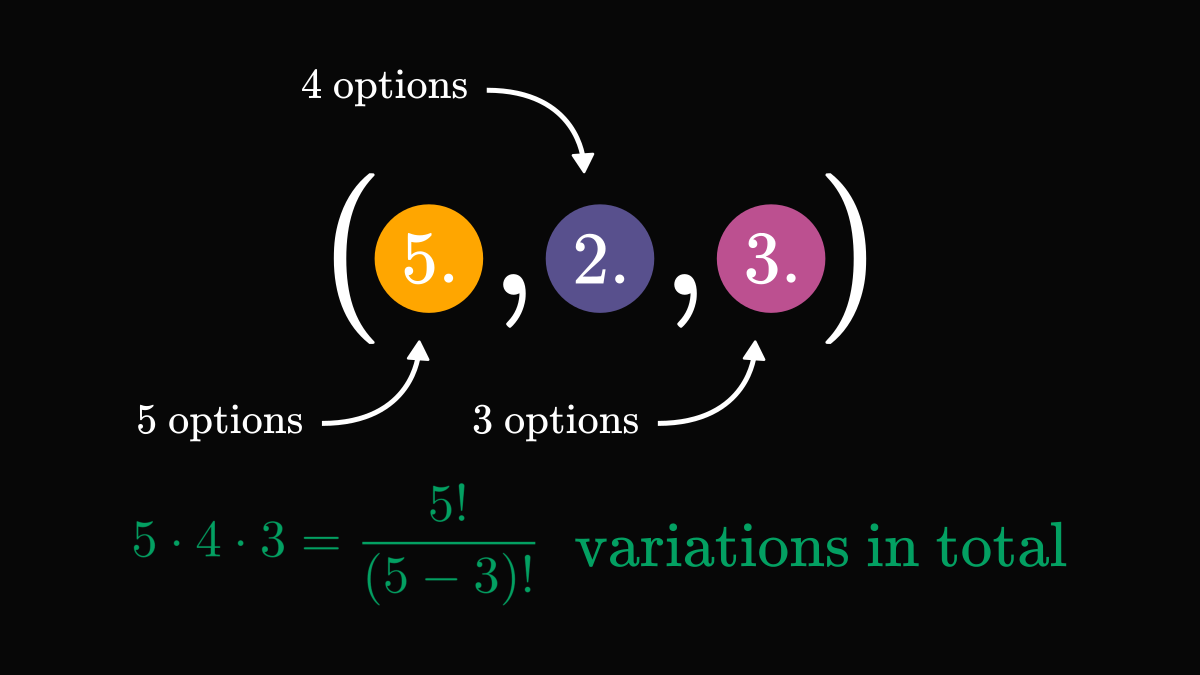Now let's count variations again, a bit differently this time!

A variation can be obtained by first selecting a subset, then putting its elements into order. As there are $3!$ orderings (= permutations) per subset and $\binom{5}{3}$subsets in total, we have $3! \binom{5}{3}$ variations. (The expression $\binom{n}{k}$ is read as "$\textstyle n$ over $\textstyle k$".)

If you want to visualize this process, check out this illustration below.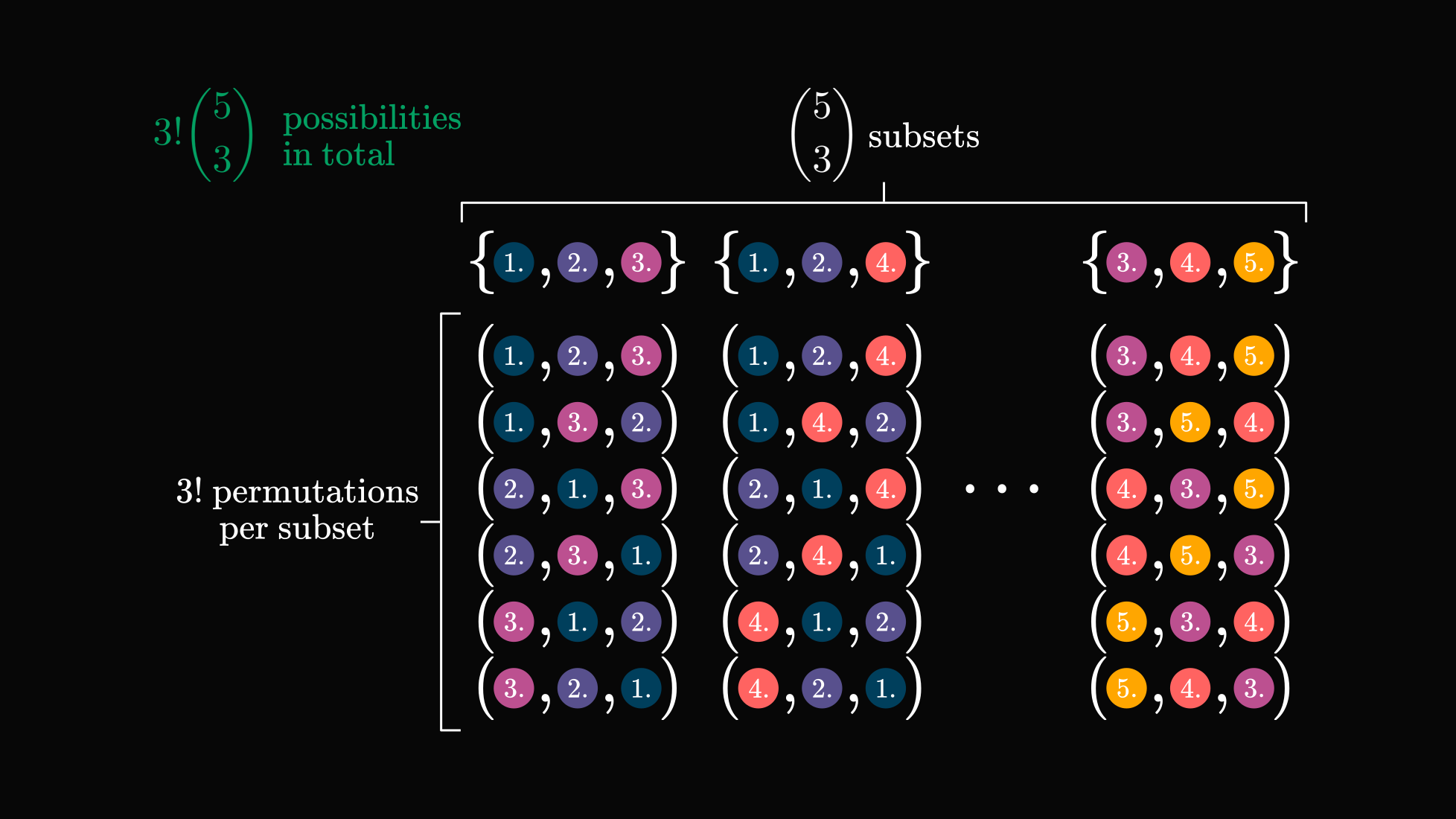Since we have counted variations in two different ways, the results must be equal. Thus, we have

$3! \binom{5}{3} = \frac{5!}{(5 - 3)!}.$

This is true in the general case as well: the number permutations times combinations equals the number of variations.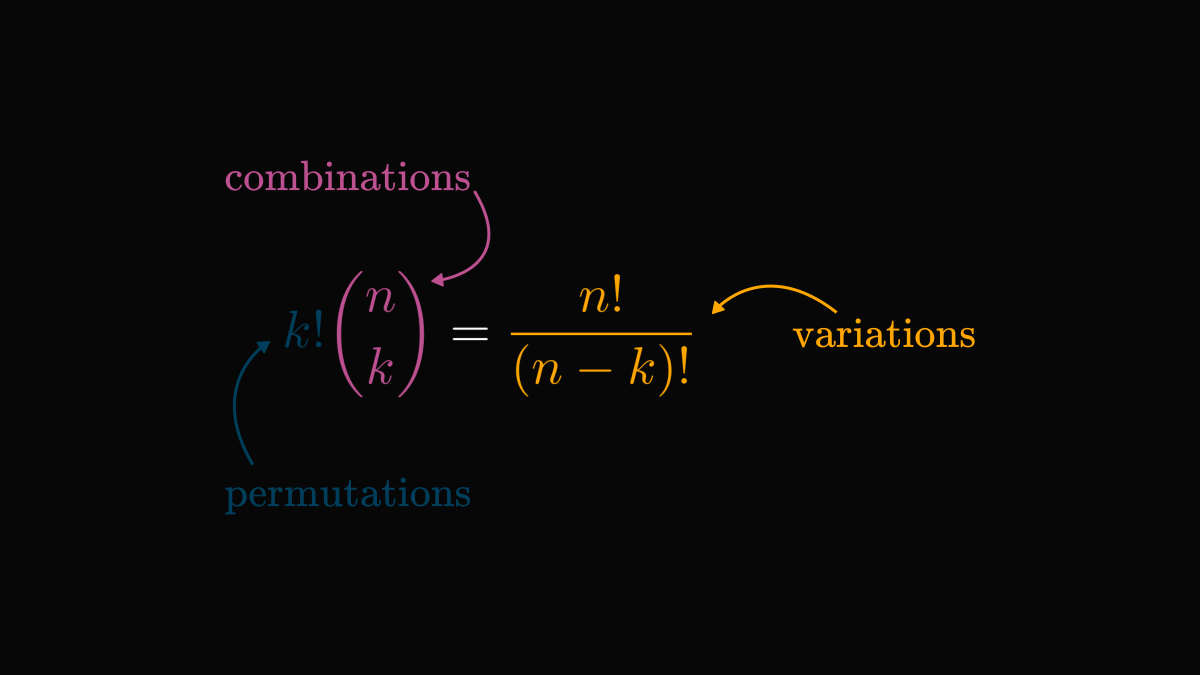By dividing with $k!$, we obtain the formula used to compute binomials. (Well, up to certain computational limitations. Factorials blow up really fast, and dividing two huge numbers is not a good idea.) That is, we have

$\binom{n}{k} = \frac{n!}{k! (n - k)!}.$

So, why is the combinatorial definition useful?

For one, think about the famous binomial theorem:

$(a + b)^n = \sum_{k=0}^{n} \binom{n}{k} a^k b^{n - k}.$

How would you prove it? The simplest way is to use combinatorics and count the number of ways each a-b term can be obtained from the product, which is given by the binomial coefficients.